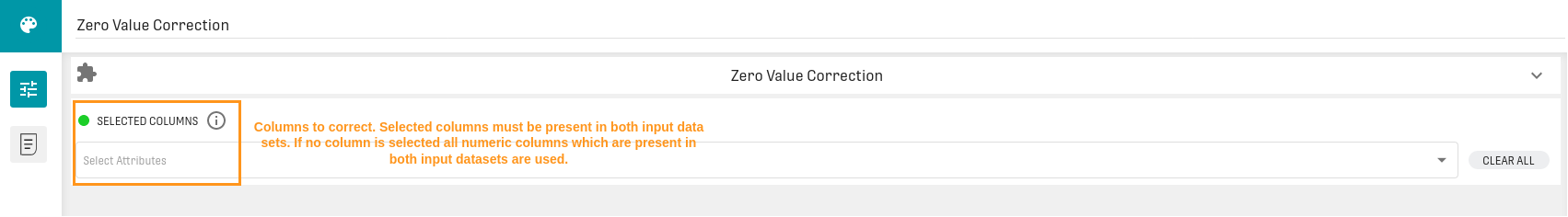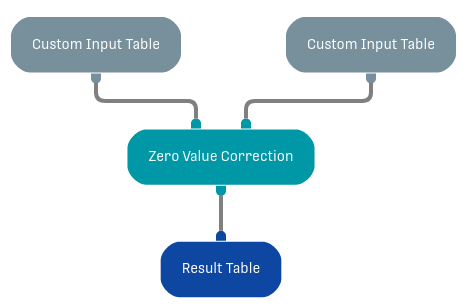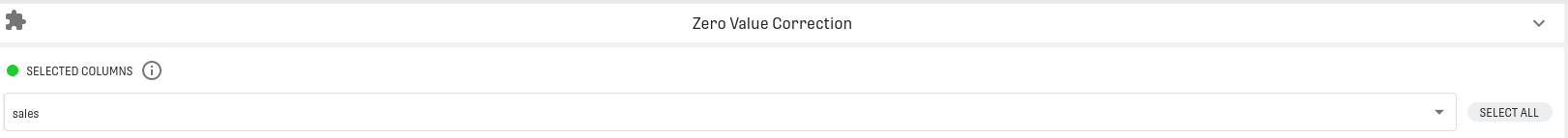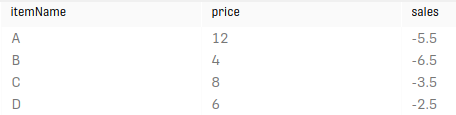# Overview

The Zero Value Correction Processor corrects the values of chosen columns by subtracting specified delta values.

# Input

The processor has two input ports. The left port is for the dataset that has to be corrected. The right port for the table containing the delta values. Note that the corresponding 'correction' and 'delta' columns have to have the same column name. Also the input columns need to have a numeric data type (int, double or numeric).

# Configuration

In the processor configuration, the columns to correct can be specified.# Output

The output of the processor is the corrected dataset.

The delta value is always the average value of the according column in the right input node.

# Example

In this example we use a Custom Input Table Processor to create an artificial sales dataset which is given below. Then the Zero Value Correction Processor is used to subtract the mean from each of the values of the dataset.

## Workflow## Configuration## ResultIn the output of the Zero Value Correction Processor the mean of all values of the right input dataset's sales  (in this case 6.5) was subtracted of all values of the left input dataset.
So the result in the first row of the output is calculated by: 1 - 6.5 = -5.5
The result of the second row of the output is calculated by: 0 - 6.5 = -6.5
And so on for the further rows.